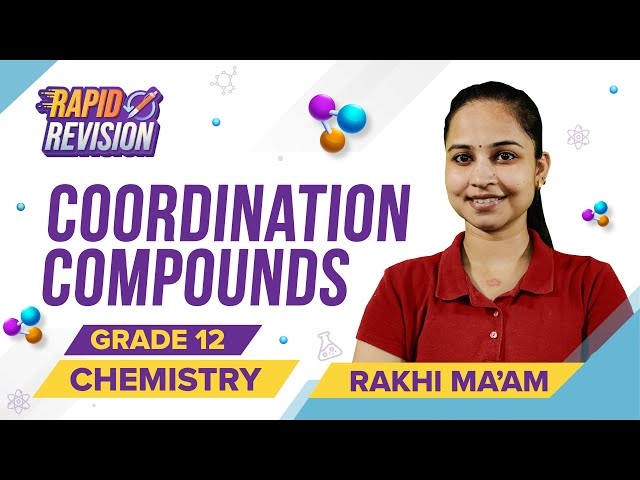Win up to 100% scholarship on Aakash BYJU'S JEE/NEET courses with ABNAT Win up to 100% scholarship on Aakash BYJU'S JEE/NEET courses with ABNAT

# Coordination Compounds ‌–‌ ‌JEE‌ ‌Advanced‌ ‌Previous‌ ‌Year‌ ‌Questions with Solutions

Coordination Compounds JEE Advanced Previous Year Questions with Solutions can be accessed here. This set of questions has been taken from the previous year JEE Advanced question papers. JEE aspirants will find step-by-step explanations for the questions listed here. So the questions along with detailed solutions will really enhance the student’s grip on the topic and they will also get a better idea of the question types, difficulty level and pattern of the paper.

Alternatively, as aspirants go through the questions and solutions they will develop better skills to solve different types of problems and prepare effectively for the upcoming entrance exam. JEE aspirants may also download Coordination Compounds JEE Advanced Previous Year Questions with Solutions PDF for free and use it for offline practice.

## JEE Advanced Previous Year Questions on Coordination Compounds

Question 1. Total number of cis N–Mn–Cl bond angles (that is, Mn–N and Mn–Cl bonds in cis positions) present in a molecule of cis-[Mn(en)2Cl2] complex is _________ (en = NH2CH2CH2NH2)

Solution: (6)

This is the cis form of [Mn(en)2Cl2]

Therefore, No. of N–Mn–Cl bonds = 6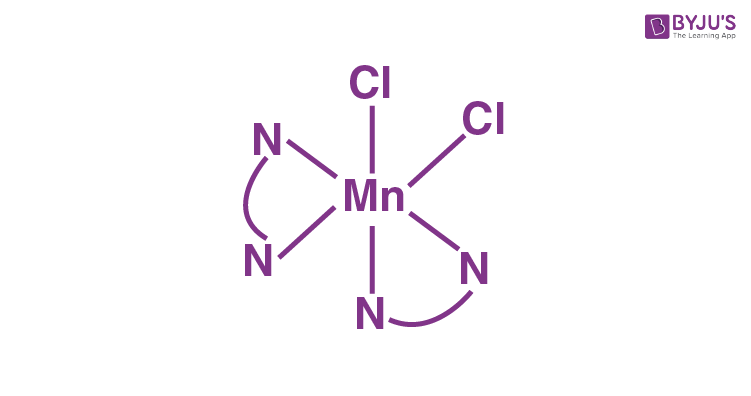Question 2. The number of geometric isomers possible for the complex [CoL2Cl2](L=H2NCH2CH2O) is:

Solution: (5)

The general form of complex is [M(AB)2a2]

The total possible geometrical isomers are 5

There are 5 geometric isomers of the complex [CoL2Cl2] as shown.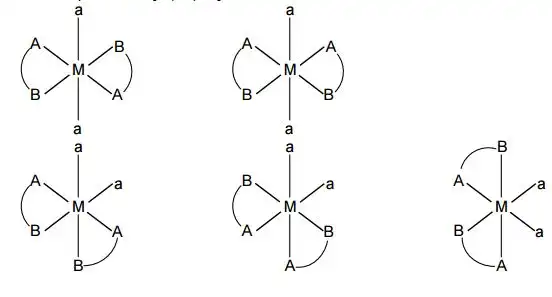Question 3. Among the complex ions, [Co(NH2.CH2.CH2.NH2)2Cl2|]+, [CrCl2(C2O4)2]3−, [Fe(H2O)4(OH)2]+, [Fe(NH3)2(CN)4], [Co(NH2− CH2− NH2)2(NH3)Cl]2+ and [Co(NH3)4(H2O)Cl]2+, the number of complex ion(s) that show(s) cis-trans isomerism is:

Solution: (6)

Basically, all the complexes given show cis-trans isomerism.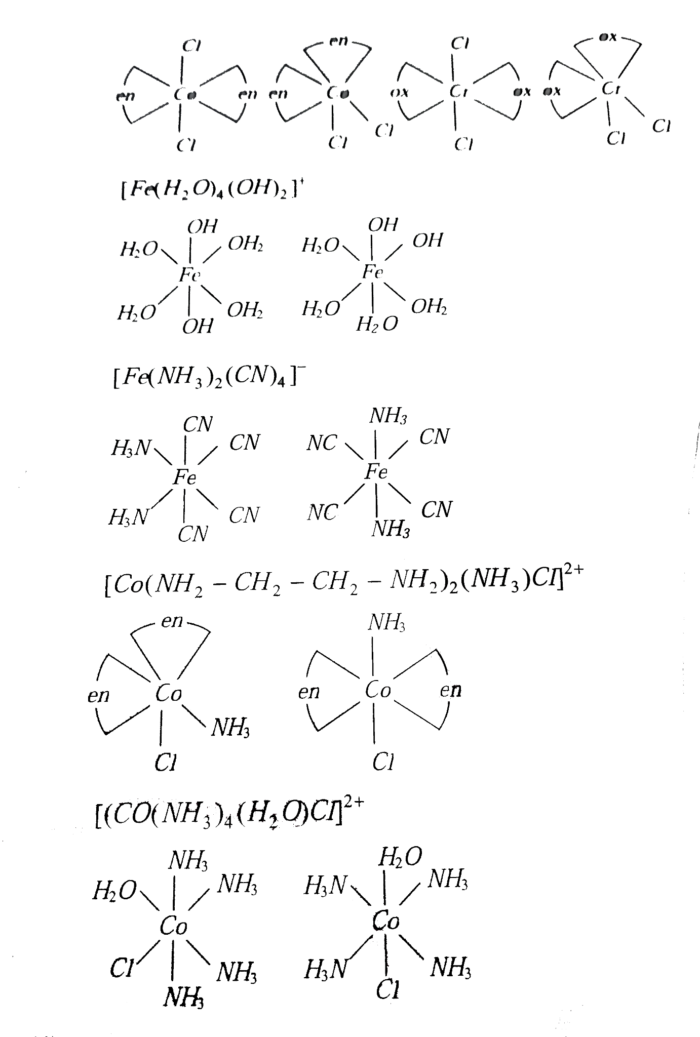Question 4. EDTA4- i9s ethylenediamine tetraacetate ion The total number of N-CO-O bond angles in [Co(EDTA)]-1 complex ion is.

Solution: (8)

DTA is a multidentate ligand as it can donate six pairs of electrons – two pairs, from the two nitrogen atoms and four pairs from the four-terminal oxygen of the -COO groups.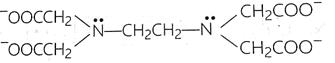The structure of a chelate of a divalent

Co3+ with EDTA is shown as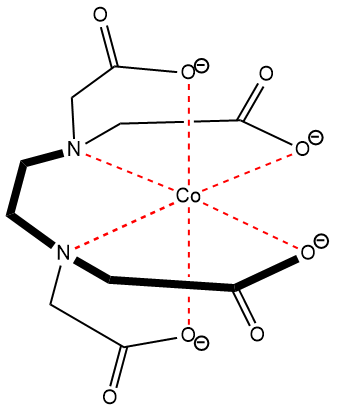Each N has four N–Co–O bonds thus a total of eight N–Co–O bonds.

Question 5. The pair(s) of coordination complexes/ions exhibiting the same kind of isomerism is/are

A. [Cr(NH3)5Cl]Cl2 and [Cr(NH3)4Cl2]Cl

B. [Co(NH3)4Cl2]+ and [Pt(NH3)2(H2O)Cl]+

C. [CoBr2Cl2]2− and [PtBr2Cl2]2−

D. [Pt(NH3)3(NO3)]Cl and [Pt(NH3)3Cl]Br

Solution: (B and D)

Octahedral [Co(NH3)4Cl2]+ and square planar [Pt(NH3)2(H2O)Cl]+ which shows geometrical (cis-trans) isomerism.

Square planar [Pt(NH3)3(NO3)]Cl and square planar [Pt(NH3)3Cl]Br shows ionization isomerism.

Question 6. The geometries of the ammonia complexes of Ni2+, Pt2+ and Zn2+, respcetively, are

A. octahedral, square planar and tetrahedral

B. square planar, octahedral and tetrahedral

C. tetrahedral, square planar and octahedral

D. octahedral, tetrahedral and square planar

Solution: (A)

Ni2+ → Octahedral → [Ni(NH3)6]2+

Pt2+ → sq. Planar → [Pt(NH3)4]2+

Zn2+ → Tetrahedral → [Zn(NH3)4]2+

Question 7. Consider the following complex ions, P,Q and R.

P = [FeF6]3−,Q = [V(H2O)6]2+ and R = [Fe(H2O)6]2+

The correct order of the complex ions, according to their spin-only magnetic moment values (in B.M.) is

A. R < Q < P

B. Q < R < P

C. R < P < Q

D. Q < P < R

Solution: (B)

The correct option is B. Q < R < P

μspin only = √n(n + 2)

Magnetic moment arises due to the presence of unpaired electrons.

More the number of unpaired electrons, greater will be the magnetic moment.

P has Fe in +3 oxidation state.

Fe(+3) : 3d5

Q has V in +2 oxidation state.

V(+2) : 3d3

R has Fe in +2 oxidation state.

Fe(+2) : 3d6

Hence the number of unpaired electrons in P,Q and R are 5,3 and 4 respectively.

Question 8. Among the species given below, the total number of diamagnetic species is____. H atom, NO2 monomer, O2– (superoxide), dimeric sulphur in vapour phase, Mn3O4, (NH4)2[FeCl4], (NH4)2[NiCl4], K2MnO4, K2CrO4.

Solution:

Paramagnetic: H,NO2 monomer, O2 (superoxide), S2 (Vapour), [Mn3O4 is mixed oxide of Mn+2 and Mn+3], (NH4)2[FeCl4], (NH4)2[NiCl4], K2MnO4

Diamagnetic: K2CrO4

Question 9. For the octahedral complexes of Fe3+ in SCN (thiocyanato-S) and in CN ligand environments, the difference between the spin-only magnetic moments in Bohr magnetons (when approximated to the nearest integer) is ____. [Atomic number of Fe = 26]

Solution: (√35 − √3 ≃ 4)

SCN is a weak field effect (WFE) ligand whereas CN is a strong field effect (SFE) ligand.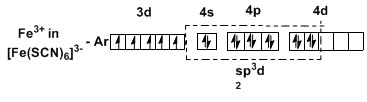Spin only magnetic moment = √5(5 + 2) = √35 B.M.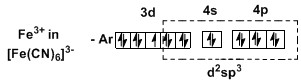Spin only magnetic moment =​ ​√1(1 + 2) = √3 B.M.

BM Difference =​ ​√35 − √3 ≃ 4

Question 10. Choose the correct statement(s) among the following:

A. [FeCl4] has tetrahedral geometry

B. [Co(en)(NH3)2Cl2]+ has 2 geometrical isomers

C. [FeCl4] has higher spin-only magnetic moment than [Co(en)(NH3)2Cl2]+

D. The cobalt ion in [Co(en)(NH3)2Cl2]+ has sp3d2 hybridization

Solution: (A and C)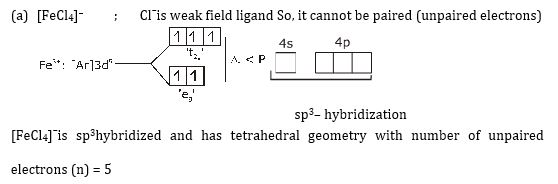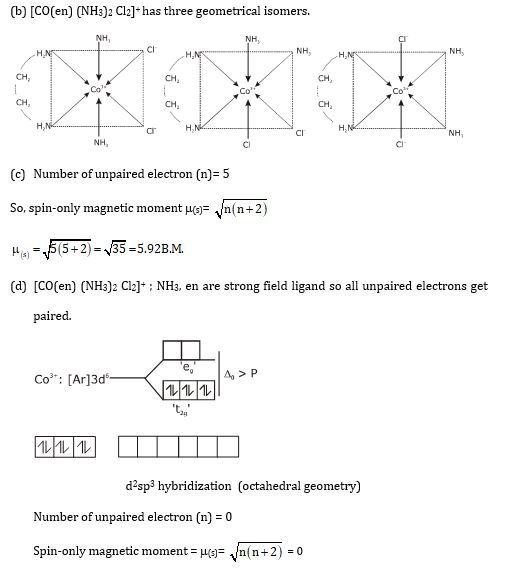Question 11. Match each set of hybrid orbitals from LIST -I was complex(es given in LIST – II.

List-I List-II
P. dsp2 1. [FeF6]4-
Q. sp3 2 [Ti(H2O)3Cl3]
R. sp3d2 3 [Cr(NH3)6]3+
S. d2sp3 4 [FeCl4]2-
5 Ni(CO)4
6 [Ni(CN)4]2-

A. P → 5; Q → 4,6; R → 2,3; S → 1

B. P → 5,6; Q → 4; R → 3; S → 1,2

C. P → 6; Q → 4,5; R → 1; S → 2,3

D. P → 4,6; Q → 5,6; R → 1,2; S → 3

Solution: (C)

dsp2: [Ni(CN)4]2-

sp3: [FeCl4]2-; Ni(CO)4

sp3d2: [FeF6]4-

d2sp3: [Ti(H2O)3Cl3], [Cr(NH3)6]3+

## Coordination Compounds – Rapid Revision